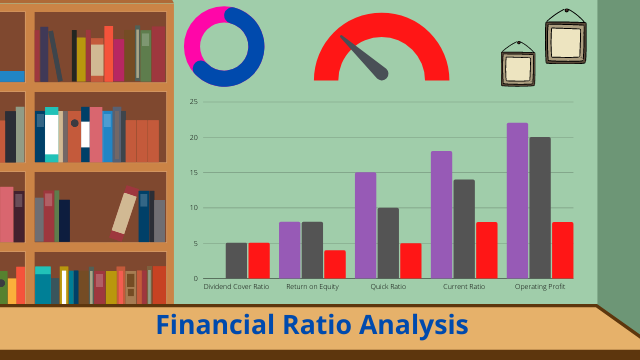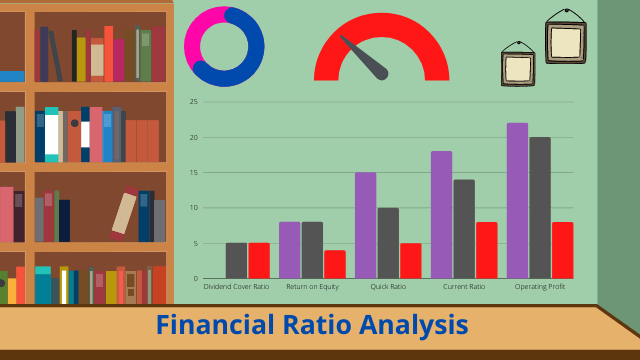# Financial Ratio Analysis## Financial Ratio Analysis

Introduction – Financial Ratio Analysis

Financial ratio analysis are very helpful for taking decision regarding the choice of appropriate project for investment as there are so many financial factors are related (Foerster, 2015) . The firm is financially sound or not, financial performance is good or bad, debt is high or low, profit margin s high or low, each and every factors financial statements could be utilized by using financial ratio analysis. This study will focus on ratio analysis between Jones Ltd and Millet Ltd with recommendation to choose a project between this two. Even, this study will also focus on the cost classification in the production of goods and services.

1. Ratio Analysis

Company: Jones Ltd

1. Operating Profit Margin

Formula = (Operating Profit / Sales Revenue) X 100

= (5970 / 25160) X 100

= 23.73%

1. Return on Shareholder’s Fund

Formula = (Profit after tax / Shareholder’s capital) X 100

= (4710 / 11000) X 100

= 42.82%

1. Return on Equity

Formula = (Profit after tax / Shareholder’s equity) X 100

= (4710 / 15092) X 100

= 31.21%

1. Inventory Turnover

Formula = (Cost of Goods sold / Inventory)

= (15720 / 1730)

= 9.09 times

1. Accounts Receivable Collection Period

Formula = (365 / Account Receivable Turnover Ratio)

= (365/27.71)

= 13.18 days

Here, Account Receivable Turnover Ratio = (Account Receivable / Net Credit Sales)

= (1910 / 68.93)

= 27.71 times

Here, Net Credit Sales = (25160 / 365)

= 68.93

1. Quick ratio

Formula = (Current Asset-Inventories) / Current Liabilities

= (4337-1730) / 3265

= 0.79:1

1. Gearing Ratio

Formula = (Total Debt/Total Equity) X 100

= (7365 / 15092) X 100

= 48.80%

1. Interest Cover Ratio

Formula = (EBIT / Interest Expense)

= (5970 / 670)

= 8.91 times

1. Dividend Cover Ratio

Formula = (Net Income / Dividend)

= (4710 / 490)

= 9.61 times

1. Dividend Per Share

Formula = (Dividends / Number of Share Outstanding)

= (490 / 11000)

= 0.044

Here, Number of share outstanding = (Share Capital / Share Price)

= (11000 / 1)

= 11000

Company: Millet Ltd

1. Operating Profit Margin

Formula = (Operating Profit / Sales Revenue) X 100

= (5260 / 20260) X 100

= 25.96%

1. Return on Shareholder’s Fund

Formula = (Profit after tax / Shareholder’s capital) X 100

= (4530 / 11000) X 100

= 41.18%

1. Return on Equity

Formula = (Profit after tax / Shareholder’s equity) X 100

= (4530 / 14590) X 100

= 31.05%

1. Inventory Turnover

Formula = (Cost of Goods sold / Inventory)

= (12680 / 1370)

= 9.25 times

1. Accounts Receivable Collection Period

Formula = (365 / Account Receivable Turnover Ratio)

= (365 / 25.76)

= 14.17 days

Here, Account Receivable Turnover Ratio = (Account Receivable / Net Credit Sales)

= (1430 / 55.51)

= 25.76 times

Here, Net Credit Sales = (20260 / 365)

= 55.51

1. Quick ratio

Formula = (Current Asset – Inventories) / Current Liabilities

= (3890 – 1370) / 2080

= 1.21: 1

1. Gearing Ratio

Formula = (Total Debt / Total Equity) X 100

= (2780 / 14590) X 100

= 19.05%

1. Interest Cover Ratio

Formula = (EBIT / Interest Expense)

= (5260 / 120)

= 43.83 times

1. Dividend Cover Ratio

Formula = (Net Income / Dividend)

= (4530 / 820)

= 5.52 times

1. Dividend Per Share

Formula = (Dividends / Number of Share Outstanding)

= (820 / 44000)

= 0.019

Here, Number of share outstanding = (Share Capital / Share Price)

= (11000 / 0.25)

= 44000

2. Operating Profit Margin Ratio

This ratio basically represents the operating income in terms of profitability of the business (Singh, 2017). From the above results, it is highlighted that operating profit margin ratio of Jones Ltd is 23.73% and operating profit margin ratio of Millet Ltd is 25.96%. When the firm has high operating margin ratio, it is considered as financially sound company. Therefore, Millet Ltd is comparatively more financially sound than Jones Ltd as it has high operating profit margin.

1. Return on Shareholder’s Fund

This ratio basically represents the profitability of the firm whereas the firm is able to repay its shareholder’s fund investment (Singh, 2017). From the above results, it is highlighted that Return on shareholder’s fund of Jones Ltd is 42.82% and Return on shareholder’s fund of Millet Ltd is 41.18%. When the firm is able to repay its shareholder’s fund investment, the firm is financially strong and able to cover the return from investment. Therefore, Jones Ltd is comparatively more able to cover its return from investment than Millet Ltd as it has high Return on shareholder’s fund ratio.

1. Return on Equity

This ratio basically represents the financial performance of the firm whereas how effective the firm is to use its assets for earning profit (Singh, 2017). From the above results, it is highlighted that Return on equity of Jones Ltd is 31.21% and Return on equity of Millet Ltd is 31.05%. When the firm is highly effective to use its asset for generating more profit from equity investment and able to cover the return from equity investment, the financial performance of the firm is significantly good. Therefore, Jones Ltd is comparatively more able to cover its return from equity investment than Millet Ltd as it has high Return on equity ratio.

1. Inventory Turnover

This ratio basically represents how many times a firm is able to sell its inventory for a given period time (Singh, 2017). From the above results, it is highlighted that Inventory Turnover of Jones Ltd is 9.09 times and Inventory Turnover of Millet Ltd 9.25 times. When the firm is highly effective to sell its inventory at maximum times, then its helps management to take decision immediately regarding purchasing new inventory and also the cost of inventory becomes lower.  Therefore, Millet Ltd is comparatively selling more inventory for maximum times than Jones Ltd as it has high inventory turnover ratio.

1. Accounts Receivable Collection Period

This ratio basically represents collection period of credit sales for a given period time (Singh, 2017). From the above results, it is highlighted that Accounts Receivable Collection Period of Jones Ltd is 13.18 days and Accounts Receivable Collection Period of Millet Ltd is 14.17 days. When the firm is highly effective to collect its credit sales money within short time, then it is very helpful to maintain a healthy cash inflow. Therefore, Jones Ltd is comparatively taking less time to make the collection of credit sales than Millet Ltd as it has lower Accounts Receivable Collection Period.

1. Quick Ratio

This ratio basically represents the ability to pay off its short term obligations by converting marketable securities and account receivable into cash (Singh, 2017). From the above results, it is highlighted that Quick Ratio of Jones Ltd is 0.79:1 and Quick ratio of Millet Ltd is 1.12:1. When the firm is highly able to pay off its short term obligations, the firm is financially sustainable. Therefore, Millet Ltd is comparatively in good position than Jones Ltd as it has high quick ratio.

1. Gearing Ratio

This ratio basically represents the proportion of debt obligations relative to equity portion with different financial metrics (Singh, 2017). From the above results, it is highlighted that Gearing Ratio of Jones Ltd is 48.80% and Gearing ratio of Millet Ltd is 19.05%. If the firm has less debt portion then equity portion, then the firm is not financially solvent and huge debt burden. Therefore, Jones Ltd has more debt portion than Millet Ltd and Jones is not in good position.

1. Interest Cover Ratio

This ratio basically represents the ability of the firm to pay interest of the outstanding debt (Singh, 2017). From the above results, it is highlighted that Interest Cover Ratio of Jones Ltd is 8.91 times and Interest Cover ratio of Millet Ltd is 43.83 times. If the interest cover ratio is higher, it is considered as better firm who is good to pay interest maximum times. Therefore, Millet Ltd is more able to pay interest at maximum times for debt outstanding than Jones Ltd.

1. Dividend Cover Ratio

This ratio basically represents the ability of the firm to pay dividend to its shareholders (Singh, 2017). From the above results, it is highlighted that Dividend Cover Ratio of Jones Ltd is 9.61 times and Dividend Cover ratio of Millet Ltd is 5.52 times. If the dividend cover ratio is higher, it is considered as better firm who is good to satisfy its shareholders. Therefore, Millet Ltd is more able to pay dividend at maximum times than Jones Ltd.

1. Dividend Per Share

This ratio basically represents the amount of dividend attributed to each shareholders (Singh, 2017). From the above results, it is highlighted that Dividend per share of Jones Ltd is 0.044 and Dividend per share of Millet Ltd is 0.019. If the dividend per share is higher, it is considered as better firm to attract more investor to invest in the firm. Therefore, Jones Ltd is highly attributed for maximum dividend per share than Millet Ltd.Sl Financial Ratio Analysis Jones Ltd. Millet Ltd. Recommendation 01 Operating Profit Margin 23.73% 25.96% Millet Ltd. 02 Return on Shareholder’s Fund 42.82% 41.18% Jones Ltd. 03 Return on Shareholder’s equity 31.21% 31.05% Jones Ltd. 04 Inventory Turnover 9.09 times 9.25 times Millet Ltd. 05 Accounts Receivable Collection Period 13.18 days 14.17 days Jones Ltd. 06 Quick Ratio 0.79:1 1.21:1 Millet Ltd. 07 Gearing Ratio 48.80% 19.05% Millet Ltd. 08 Interest Cover Ratio 8.91 times 43.83 times Millet Ltd. 09 Dividend Cover Ratio 9.61 times 5.52 times Jones Ltd. 10 Dividend Per Share 0.044 0.019 Jones Ltd.

Recommendation

From the above table, it is very tough to recommend for Benns Ltd for investing in Jones Ltd or Millet Ltd because from the above ratios Jones is in good position with 5 ratios and even Millet Ltd is also in good position with 5 ratios.

Still, it could be recommended for Benns Ltd for investing in Jones Ltd because in terms of profitability ratios Jones is in good position because it has higher Return on Shareholder’s Fund and Return on equity. Decision taking by observing profitability ratios is always preferable because profit is the prime goal of the business that’s why Jones Ltd is the good option for Benns Ltd to invest in it.

1. Limitations of Financial Ratios in Decision Making
• Ratios are calculated from financial statements whereas the figures are subject to manipulated to some extent. In that case, it will be very tough to take right decision (Velez-Pareja, 2012).
• Ratios have the problem of comparability regarding different accounting methods like inventory turnover where FIFO method of inventory may provide one result and LIFO method may provide another result. In that case, it will be very tough to take right decision for inventory purchase (Velez-Pareja, 2012).
• Some accounting methods like depreciation, amortization, and treatment of extraordinary items may provide different results in some cases among the companies. In that case, it will be very tough to take right decision regarding investment (Velez-Pareja, 2012).
• As ratios have the assumption of ignoring price level changes that means it is ignoring the inflation. In that case, it will provide the real scenario of financial statement where management can take right decision (Velez-Pareja, 2012).

Written by